# Excel ROUNDUP Function

This post will guide you how to use Excel ROUNDUP function with syntax and examples in Microsoft excel.

### Description

The Excel ROUNDUP function rounds the number up to a specified number of decimal places. It will round away from 0.

The ROUNDUP function is a build-in function in Microsoft Excel and it is categorized as a Math and Trigonometry Function.

The ROUNDUP function is available in Excel 2016, Excel 2013, Excel 2010, Excel 2007, Excel 2003, Excel XP, Excel 2000, Excel 2011 for Mac.

### Syntax

The syntax of the ROUNDUP function is as below:

`=ROUNDUP (number, num_digits)`

Where the ROUNDUP function arguments are:

• number -This is a required argument. Any real number that you want to round up.
• num_digits – This is a required argument. The number of digits to which number you want to round up.

Note:

• The behaves of ROUNDUP like the ROUND function, the only different is that it always rounds a number up.
• If num_digits argument is greater than 0, then the number is rounded up to the specified number of digits. So it will round up to the right of the decimal point.
• If num_digits argument is equal to 0, then the number is rounded up to the nearest integer.
• If num_digits argument is less than 0, then the number is rounded up to the left of the decimal point.

### Excel ROUNDUP Function Examples

The below examples will show you how to use Excel ROUNDUP Function to round up a number away from 0.

1# to round a number 4.5 up to zero decimal places, enter the following formula in Cell B1.

`=ROUNDUP(4.5,0)`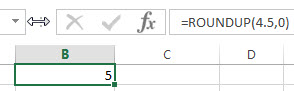2# to round a number 42.5 up to zero decimal places, enter the following formula in Cell B2.

`=ROUNDUP(42.5,0)`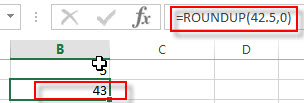3# to round a number 5.432 up to two decimal places, enter the following formula in Cell B3.

`=ROUNDUP(5.432,2)`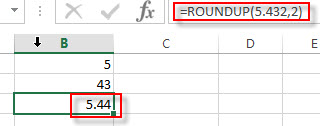4# to round a number -5.432 up to one decimal places, enter the following formula in Cell B4.

`=ROUNDUP(-5.432,1)`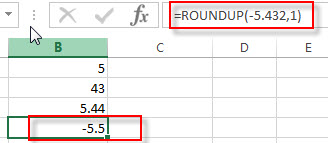5# to round a number 4324.632 up to two decimal places to the left of the decimal point, enter the following formula in Cell B5.

`=ROUNDUP(4324.632,-2)`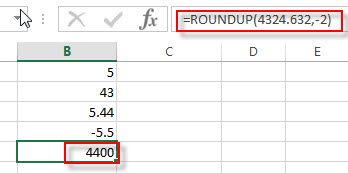### Related Functions

• Excel ROUNDDOWN function
The Excel ROUNDDOWN function round the number down to the specified number of digits. The syntax of the ROUNDDOWN function is as below:=ROUNDDOWN (number, num_digits)…
• Excel ROUND function
The Excel ROUND function rounds a number to a specified number of digits. You can use the ROUND function to round to the left or right of the decimal point in Excel.The syntax of the ROUND function is as below:=ROUND (number, num_digits)…
• Excel CEILING Function
The Excel CEILING function returns a given number rounded up to the nearest multiple of a given number of significance. The syntax of the CEILING function is as below:= CEILING (number, significance)…
• Excel CEILING.PRECISE Function
The Excel CEILING.PRECISE function returns a given number rounded up to the nearest multiple of a given number of significance or returns a number that is rounded up to the nearest integer number.The syntax of the CEILING.PRECISE function is as below:=CEILING.PRECISE (number, [significance])…